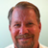# Plot Specific Lines on Lower Study Depending on Input or Stock Symbol?

T

##### Active member
2019 Donor
VIP
I'm not a programmer and tried to find this by searching but I'm not getting much results so I figured I'll just ask here since some of you seem to be so knowledgeable.

I want to plot certain items depending on the input settings or the underlying symbol. For example, I want to plot the zero line plus lines at -10, -5, -1, 1, 5, 10 as default or when I'm charting ES but when I'm charting CL, I want lines plotted for -1.5, -1, -0.5, 0.5, 1, 1.5 instead. So instead of creating a different study for each asset, I was hoping to have it in the same study but have it dynamically set depending on the symbol selected.

My search points me to the switch and case functions but I'm not sure how to proceed. This would save some time if it can be done because there are studies where the settings are specific to certain symbol or even time-frame. Can it also be set according to time-frame/tick/ATR?

T

#### tomsk

##### Well-known member
VIP
Quite straightforward, here's a study I crafted for you. I have added hooks for any different levels you might like for different symbols like /ZB, AAPL, etc, etc

Code:
``````declare lower;

def posL3;
def posL2;
def posL1;
def negL1;
def negL2;
def negL3;

if GetSymbol() == "/ES:XCME" {
posL3 = 10;
posL2 = 5;
posL1 = 1;
negL1 = -1;
negL2 = -5;
negL3 = -10;
}
else if GetSymbol() == "/CL:XNYM" {
posL3 = 1.5;
posL2 = 1;
posL1 = 0.5;
negL1 = -0.5;
negL2 = -1;
negL3 = -1.5;
}
else {
posL3 = 15;
posL2 = 10;
posL1 = 5;
negL1 = -5;
negL2 = -10;
negL3 = -15;
}

AddLabel(1, "Symbol = " + GetSymbol(), Color.Yellow);

plot posL3_ = posL3;
plot posL2_ = posL2;
plot posL1_ = posL1;
plot zero = 0;
plot negL1_ = negL1;
plot negL2_ = negL2;
plot negL3_ = negL3;
# END CODE``````

•BenTen
T

#### tomsk

##### Well-known member
VIP
Since there is symmetry between the positive and negative values, here's another version that reduces the number of levels you need to define. Either way this should get you started to what you seek to achieve

Code:
``````declare lower;

def posL3;
def posL2;
def posL1;
def negL1;
def negL2;
def negL3;

if GetSymbol() == "/ES:XCME" {
posL3 = 10;
posL2 = 5;
posL1 = 1;
negL1 = -(posL1);
negL2 = -(posL2);
negL3 = -(posL3);
}
else if GetSymbol() == "/CL:XNYM" {
posL3 = 1.5;
posL2 = 1;
posL1 = 0.5;
negL1 = -(posL1);
negL2 = -(posL2);
negL3 = -(posL3);
}
else {
posL3 = 15;
posL2 = 10;
posL1 = 5;
negL1 = -(posL1);
negL2 = -(posL2);
negL3 = -(posL3);
}

AddLabel(1, "Symbol = " + GetSymbol(), Color.Yellow);

plot posL3_ = posL3;
plot posL2_ = posL2;
plot posL1_ = posL1;
plot zero = 0;
plot negL1_ = negL1;
plot negL2_ = negL2;
plot negL3_ = negL3;``````

T

##### Active member
2019 Donor
VIP
Wow, that was quick! Thanks so much. I'll play around with the code you posted and see if I get what I need. Thanks again!Referencing plot color Questions 5Plot ArrowDown / ArrowUp followed by AddOrder blanks out the arrows Questions 9